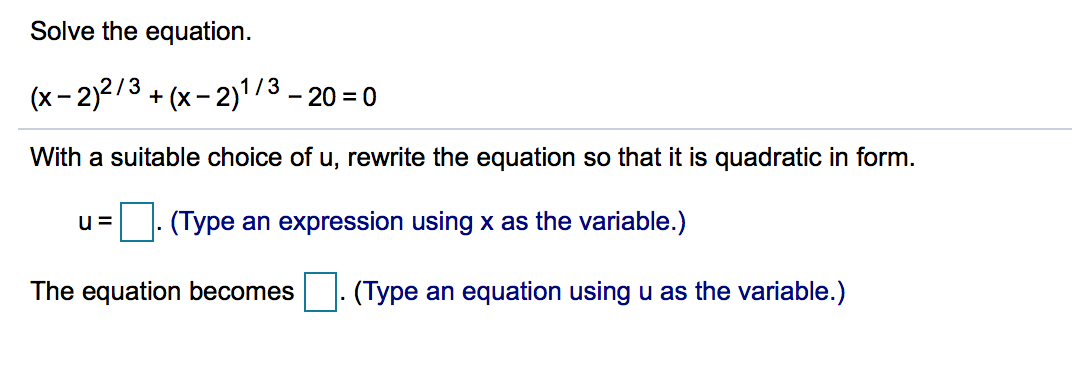# Solve the equation (x-2)2/3+(x-213-20-0 With a suitable choice of u, rewrite the equation so that it is quadratic in form. u(Type an expression using x as the variable.) The equation becomes(Type an equation using u as the variable.)

Questionhelp_outlineImage TranscriptioncloseSolve the equation (x-2)2/3+(x-213-20-0 With a suitable choice of u, rewrite the equation so that it is quadratic in form. u(Type an expression using x as the variable.) The equation becomes(Type an equation using u as the variable.) fullscreen

1 Rating

### Want to see the step-by-step answer?